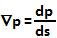## Friday, May 29, 2009

### Irodov Problem 1.325

Eulerian equation is a very general equation. There are three basic assumptions that restrict Eulerian equation into Bernoulli's equation. i) the fluid must be non-rotational (there should be no vorticity), or mathematically, ii) the force f must be a conservative force field in other words sum of potential energy and kinetic energy is conserved (such as gravity), and iii) the fluid must be incompressible (water can be considered incompressible for most practical purposes).

In order for the flow to be non-rotational any pressure change can only occur along the direction of the flow (otherwise a rotation will be induced - this not very different from if you apply force in a direction not aligned with velocity of a particle its path will exhibit curvature), in other words the direction of the gradient of pressuremust coincide with the direction of the flow of the fluid orwhere s is along the flow direction of the fluid.

If the only force acting is gravity thenalong the z-axis, the negative sign comes because gravity acts aong the negative z -axis.